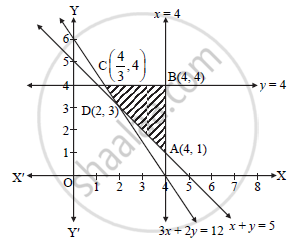# Minimize : Z = 6x + 4y Subject to the conditions: 3x + 2y ≥ 12, x + y ≥ 5, 0 ≤ x ≤ 4, 0 ≤ y ≤ 4 - Mathematics and Statistics

Sum

Minimize : Z = 6x + 4y

Subject to the conditions:

3x + 2y ≥ 12,

x + y ≥ 5,

0 ≤ x ≤ 4,

0 ≤ y ≤ 4

#### Solution

To draw feasible region, construct table as follows:

 Inequality 3x+2y ≥12 x+y ≥ 5 x ≤ 4 y ≤ 4 Correspondingequation (of line) 3x + 2y = 12 x+y=5 x=4 y=4 Intersection of linewith X-axis (4, 0) (5, 0) (4, 0) - Intersection of linewith Y-axis (0, 6) (0, 5) - (0, 4) Region Non-origin side Non-origin side Origin side Origin sideShaded portion is the feasible region,

Where B(4, 4), A(4, 1), C(4/3 , 4)

For D : 3x + 2y = 12

x + y = 5

Multiply equation (ii) by ‘2’ and subtract it from (i),

3x + 2y = 12

2x + 2y = 10

(-      -       -)/(x   = 2)

∴ y = 3 and hence D (2,3)

Z = 6x + 4y

Z at A (4,1) = 6(4) + 4(1) = 28

Z at B(4,4) = 6(4) + 4(4) = 40

Z at C (4/3 , 4) = 6 (4/3) + 4(4) = 28

Z at D (2,3) = 6(2) + 4(3) = 24

Thus, Z is minimized at D (2,3) and its minimum value is 24.

Concept: Graphical Method of Solving Linear Programming Problems
Is there an error in this question or solution?
2014-2015 (October)

Share